Home MATHEMATICS TOPIC 2: FRACTIONS ~ MATHEMATICS FORM 1

TOPIC 2: FRACTIONS ~ MATHEMATICS FORM 1

94
14
SHAREFRACTIONS ~ MATHEMATICS FORM 1

A fraction is a number which is expressed in the form of a/b where a – is the top number called numerator and b– is the bottom number called denominator.
A Fraction
Describe a fraction

FRACTIONS ~ MATHEMATICS FORM 1

A fraction is a number which is expressed in the form of a/b where a – is the top number called numerator and b– is the bottom number called denominator.

Consider the diagram below
The shaded part in the diagram above is 1 out of 8, hence mathematically it is written as 1/8Example 1
(a) 3 out of 5 ( three-fifths) = 3/5
Example 2
(b) 7 0ut of 8 ( i.e seven-eighths) = 7/8
Example 3
1. 5/12=(5 X 3)/(12 x 3) =15/36
2. 3/8 =(3 x 2)/(8 X 2) = 6/16

FRACTIONS ~ MATHEMATICS FORM 1

Dividing the numerator and denominator by the same number (This method is used to simplify the fraction)

Difference between Proper, Improper Fractions and Mixed Numbers
Distinguish proper, improper fractions and mixed numbers
Proper fraction –is a fraction in which the numerator is less than denominator

FRACTIONS ~ MATHEMATICS FORM 1

Example 4

4/5, 1/2, 11/13
Improper fraction -is a fraction whose numerator is greater than the denominator
Example 5
12/7, 4/3, 65/56
Mixed fraction –is a fraction which consist of a whole number and a proper fraction
Example 6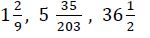(a) To convert mixed fractions into improper fractions, use the formula below
(b)To convert improper fractions into mixed fractions, divide the numerator by the denominatorExample 7
Convert the following mixed numbers into improper fractionsOperations on fractions involves addition, subtraction, multiplication and division

Addition and subtraction of fractions is done by putting both fractions under the same denominator and then add or subtract

Multiplication
of fractions is done by multiplying the numerator of the first fraction
with the numerator of the second fraction, and the denominator of the
first fraction with the denominator the second fraction.

For mixed fractions, convert them first into improper fractions and then multiply

Division of fractions is done by taking the first fraction and then multiply with the reciprocal of the second fraction

For mixed fractions, convert them first into improper fractions and then divide

FRACTIONS ~ MATHEMATICS FORM 1

Example 11

Find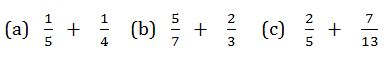Solution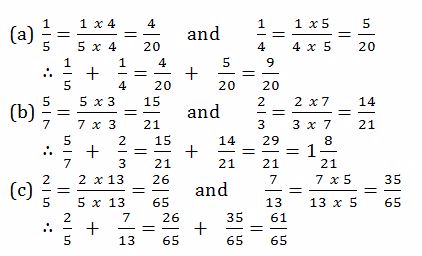Subtraction of Fractions
Subtract fractions
Example 12
Evaluate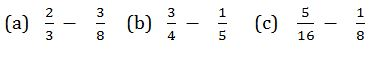Solution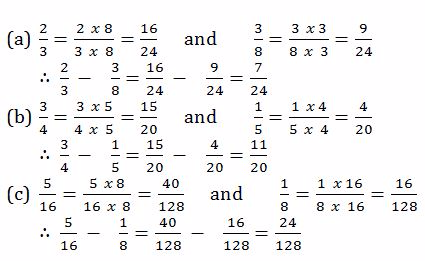Multiplication of Fractions
Multiply fractions

FRACTIONS ~ MATHEMATICS FORM 1

Example 13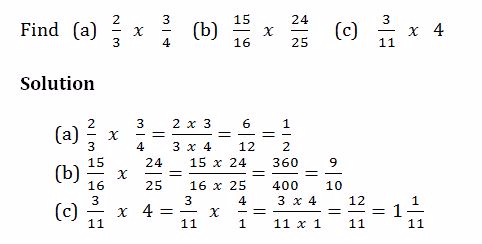Division of Fractions
Divide fractions
Example 14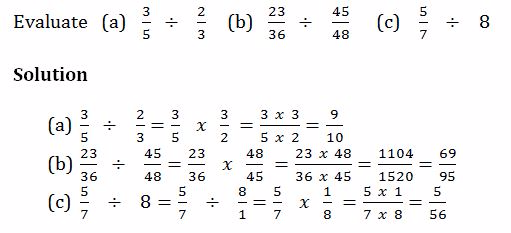Mixed Operations on Fractions
Perform mixed operations on fractions

FRACTIONS ~ MATHEMATICS FORM 1

Example 15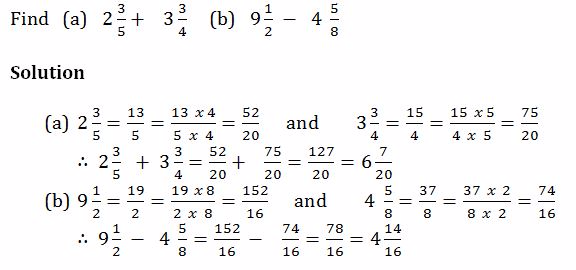Example 16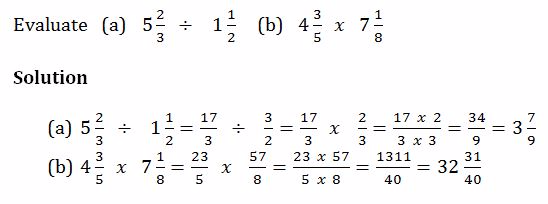Word Problems Involving Fractions
Solve word problems involving fractions

FRACTIONS ~ MATHEMATICS FORM 1

Example 17

1. Musa is years old. His father is 3¾times as old as he is. How old is his father?
2. 1¾of a material are needed to make suit. How many suits can be made from

FRACTIONS ~ MATHEMATICS FORM 1

SHARE
Previous articleTOPIC 1: NUMBERS ~ MATHEMATICS FORM 1

1. What’s up, everything is going nicely here and ofcourse every one is sharing data, that’s
in fact good, keep up writing.

2. Its like you learn my mind! You seem to understand so much approximately this, such as you wrote the guide
in it or something. I feel that you could do with some p.c.
to pressure the message house a bit, but other than that,
that is great blog. A fantastic read. I’ll certainly be back.

3. Do you have a spam problem on this site; I also am a blogger, and
I was wondering your situation; many of us have created
some nice methods and we are looking to exchange methods with other folks,
why not shoot me an e-mail if interested.

4. Wow, that’s what I was searching for, what a data!
present here at this website, thanks admin of this web page.
0mniartist asmr

5. With havin so much content do you ever run into any issues of plagorism or copyright violation? My website has
a lot of unique content I’ve either authored myself or outsourced but it appears a lot of it is popping
it up all over the internet without my permission. Do you know any
solutions to help reduce content from being
stolen? I’d truly appreciate it. 0mniartist asmr

6. Hi there mates, its enormous piece of writing about cultureand entirely explained, keep it up all the time.
asmr 0mniartist

7. Nice post. I learn something totally new and challenging on blogs I stumbleupon everyday.
It’s always exciting to read content from other authors
and use a little something from their sites. asmr 0mniartist

8. Hello There. I found your blog using msn. This is a really well written article.
Thanks for the post. I will definitely return. 0mniartist asmr

9. Hi there, i read your blog from time to time and i own a similar one and i was
just wondering if you get a lot of spam feedback? If so how do you reduce it, any
plugin or anything you can suggest? I get so much lately it’s driving me insane so any support is very much appreciated.
asmr 0mniartist

10. Simply wish to say your article is as astounding. The clearness in your submit is just great and that
i could suppose you are a professional on this subject.

Fine together with your permission allow me to take hold of
your feed to keep up to date with forthcoming post. Thanks one million and please carry on the rewarding work.
0mniartist asmr

11. Hiya! I know this is kinda off topic however I’d figured
I’d ask. Would you be interested in exchanging links or maybe guest authoring a blog post or vice-versa?
My blog discusses a lot of the same subjects as yours and I
feel we could greatly benefit from each other. If you’re interested feel free to send
me an email. I look forward to hearing from you! Fantastic
blog by the way! 0mniartist asmr

12. I don’t know whether it’s just me or if perhaps everybody
else experiencing problems with your site. It appears as though some of
the text on your content are running off the screen. Can someone else please comment and let me know if this is happening to
them as well? This might be a problem with my browser because I’ve had this happen previously.
Cheers 0mniartist asmr